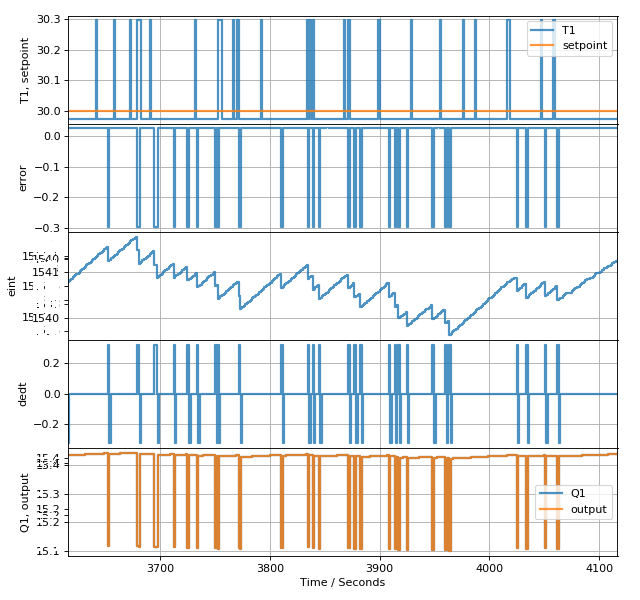# 55. PID control on TCLab¶

This notebook and the associated pidgui.py allows you to play with a very basic position form discrete PID using either a modelled version or the real Temperature control lab.

:

from tclab.gui import NotebookUI
from pidgui import PIDGUI

:

%matplotlib notebook

:

interface = NotebookUI(PIDGUI)

:

interface.gui

TCLab version 0.4.9dev
Simulated TCLab# 56. Programmatic interaction¶

We can interact with the interface while it is running. The controller is in the interface. Note that you will have to start the controller and switch to auto to see the effect of these cells:

:

controller = interface.controller

:

controller.pid.eint = 0

[ ]:

controller.setpoint.value = 42


Below we set up an experiment which will change the setpoint and increase the gain every 10 minutes.

[ ]:

import tornado

def stepgain():
if controller.setpoint.value == 45:
controller.setpoint.value = 40
else:
controller.setpoint.value = 45
controller.gain.value *= 1.1
if controller.gain.value > 100:
steptimer.stop()
interface.action_stop(None)

minute = 60*1000  # a minute in milliseconds

steptimer.start()


You can stop the timer by calling .stop()

[ ]:

steptimer.stop()


# 58. Accessing the historian¶

The interface contains a historian. You can see the sessions it has stored like this:

[ ]:

interface.historian.get_sessions()


You can roll the historian back to a session by using load_session. Note you shouldn’t do this while the interface is connected.

[ ]:

interface.historian.load_session(1)


# 59. More detailed analysis¶

We can analyse the results of the experiments we have made using Pandas:

[ ]:

import pandas

[ ]:

allresults = pandas.DataFrame.from_records(interface.historian.log, columns=interface.historian.columns, index='Time')